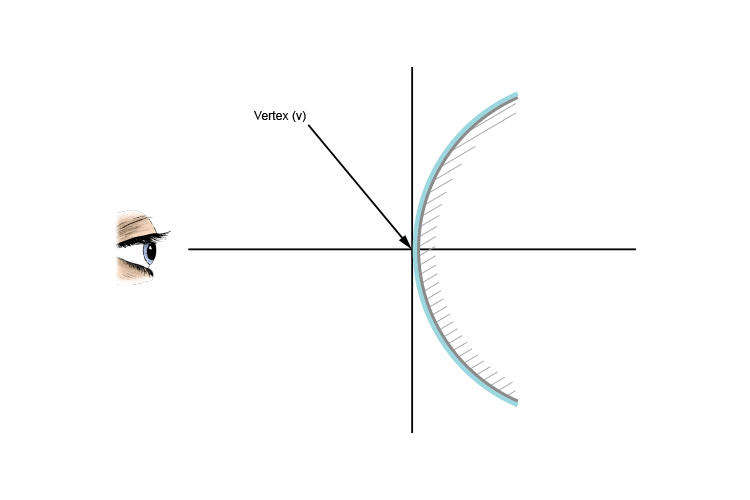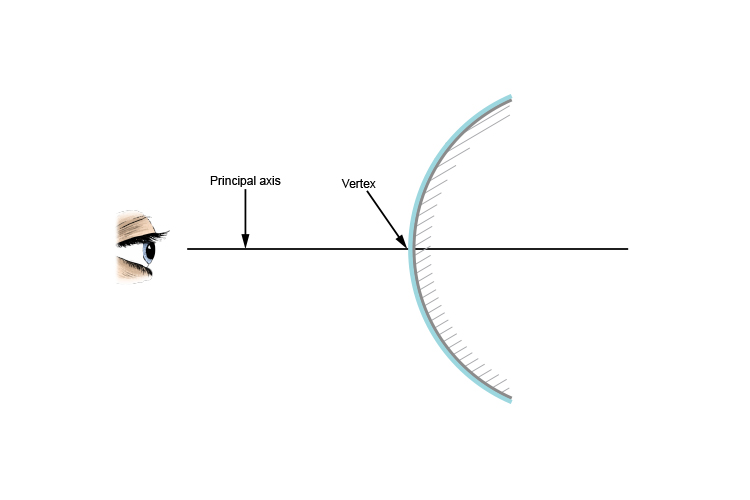# Convex mirrors and vertex

To remember the meaning of vertex:

Vertex = Vertical
and "X"= marks the spot

Vertex is nearly the word vertical and the X in the word "vertex" reminds you that it is where all the lines cross.

The convex mirror vertex is the point on the mirror's surface where the principal axis meets the mirror.

(Don't worry - "principal axis" is explained shortly!)If you draw a vertical line against the mirror, where it is just touching the mirror is the vertex.

## Convex mirror